Updating search results...

# 17 Results

View
Selected filters:
• negative-numbers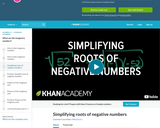Conditional Remix & Share Permitted
CC BY-NC-SA
Rating
0.0 stars

This 4-minute video lesson looks at the imaginary roots of negative numbers.

Subject:
Algebra
Mathematics
Numbers and Operations
Material Type:
Lecture
Provider:
Provider Set:
Author:
Salman Khan
10/11/2012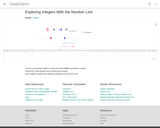Conditional Remix & Share Permitted
CC BY-NC-SA
Rating
0.0 stars

This simulation allows you to use a slider to see how positive and negative integers add on a number line.

Subject:
Algebra
Mathematics
Numbers and Operations
Material Type:
Diagram/Illustration
Simulation
Provider:
GeoGebra
Author:
rsnyder
05/10/2018Conditional Remix & Share Permitted
CC BY-NC
Rating
0.0 stars
Rating
0.0 stars
Subject:
Mathematics
Material Type:
Full Course
Provider:
Pearson
10/06/2016Conditional Remix & Share Permitted
CC BY-NC
Rating
0.0 stars

Rational Numbers

Type of Unit: Concept

Prior Knowledge

Students should be able to:

Solve problems with positive rational numbers.
Plot positive rational numbers on a number line.
Understand the equal sign.
Use the greater than and less than symbols with positive numbers (not variables) and understand their relative positions on a number line.
Recognize the first quadrant of the coordinate plane.

Lesson Flow

The first part of this unit builds on the prerequisite skills needed to develop the concept of negative numbers, the opposites of numbers, and absolute value. The unit starts with a real-world application that uses negative numbers so that students understand the need for them. The unit then introduces the idea of the opposite of a number and its absolute value and compares the difference in the definitions. The number line and positions of numbers on the number line is at the heart of the unit, including comparing positions with less than or greater than symbols.

The second part of the unit deals with the coordinate plane and extends student knowledge to all four quadrants. Students graph geometric figures on the coordinate plane and do initial calculations of distances that are a straight line. Students conclude the unit by investigating the reflections of figures across the x- and y-axes on the coordinate plane.

Subject:
Mathematics
Numbers and Operations
Material Type:
Unit of Study
Provider:
Pearson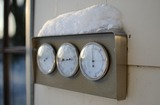Conditional Remix & Share Permitted
CC BY-NC
Rating
0.0 stars

Students answer questions about low temperatures recorded in Barrow, Alaska, to understand when to use negative numbers and when to use the absolute values of numbers.Key ConceptsThe absolute value of a number is its distance from 0 on a number line.The absolute value of a number&nbsp;n is written&nbsp;|n| and is read as &ldquo;the absolute value of n.&rdquo;A number and the opposite of the number always have the same absolute value. As shown in the diagram,&nbsp;|3| = 3 and |&minus;3| = 3.In general, taking the opposite of&nbsp;n changes the sign of n. For example, the opposite of 3 is &ndash;3.In general, taking the absolute value of&nbsp;n gives a number,&nbsp;|n|, that is always positive unless n = 0. For example,&nbsp;|3| = 3 and |&minus;3| = 3.The absolute value of 0 is 0, which is neither positive nor negative: |0| = 0.Goals and Learning ObjectivesUnderstand when to talk about a number as negative and when to talk about the absolute value of a number.Locate the absolute value of a and the absolute value of b on a number line that shows the location of a and b in different places in relation to 0.

Subject:
Numbers and Operations
Material Type:
Lesson Plan
09/21/2015Conditional Remix & Share Permitted
CC BY-NC
Rating
0.0 stars

Four full-year digital course, built from the ground up and fully-aligned to the Common Core State Standards, for 7th grade Mathematics. Created using research-based approaches to teaching and learning, the Open Access Common Core Course for Mathematics&nbsp;is designed with student-centered learning in mind, including activities for students to develop valuable 21st century skills and academic mindset.

Subject:
Mathematics
Material Type:
Full Course
Provider:
Pearson
10/06/2016Conditional Remix & Share Permitted
CC BY-NC
Rating
0.0 stars

Algebraic Reasoning

Type of Unit: Concept

Prior Knowledge

Students should be able to:

Add, subtract, multiply, and divide rational numbers.
Evaluate expressions for a value of a variable.
Use the distributive property to generate equivalent expressions including combining like terms.
Understand solving an equation or inequality as a process of answering a question: which values from a specified set, if any, make the equation or inequality true?
Write and solve equations of the form x+p=q and px=q for cases in which p, q, and x are non-negative rational numbers.
Understand and graph solutions to inequalities x&lt;c or x&gt;c.
Use equations, tables, and graphs to represent the relationship between two variables.
Relate fractions, decimals, and percents.
Solve percent problems included those involving percent of increase or percent of decrease.

Lesson Flow

This unit covers all of the Common Core State Standards for Expressions and Equations in Grade 7. Students extend what they learned in Grade 6 about evaluating expressions and using properties to write equivalent expressions. They write, evaluate, and simplify expressions that now contain both positive and negative rational numbers. They write algebraic expressions for problem situations and discuss how different equivalent expressions can be used to represent different ways of solving the same problem. They make connections between various forms of rational numbers. Students apply what they learned in Grade 6 about solving equations such as x+2=6 or 3x=12 to solving equations such as 3x+6=12 and 3(x−2)=12. Students solve these equations using formal algebraic methods. The numbers in these equations can now be rational numbers. They use estimation and mental math to estimate solutions. They learn how solving linear inequalities differs from solving linear equations and then they solve and graph linear inequalities such as −3x+4&lt;12. Students use inequalities to solve real-world problems, solving the problem first by arithmetic and then by writing and solving an inequality. They see that the solution of the algebraic inequality may differ from the solution to the problem.

Subject:
Algebra
Mathematics
Material Type:
Unit of Study
Provider:
PearsonConditional Remix & Share Permitted
CC BY-NC
Rating
0.0 stars

Students discover how the addition and multiplication properties of inequality differ from the addition and multiplication properties of equality.Students use the addition and multiplication properties of inequality to solve inequalities. They graph their solutions on the number line.Key ConceptsIn this lesson, students extend their knowledge of inequalities from Grade 6. In Grade 6, students learned that solving an inequality meant finding which values made the inequality true. Students used substitution to determine whether a given value made an inequality true. They also used a number line to graph the solutions of inequalities. By graphing these solutions on a number line, they saw that an inequality has an infinite number of solutions.Now, in Grade 7, students work with inequalities that also contain negative numbers and learn to solve and graph solutions for inequalities such as &minus;2x &minus; 4 &lt; 5. This involves first understanding how the properties of inequality differ from the properties of equality. When multiplying (or dividing) both sides of an inequality by the same negative number, the relationship between the two sides of the inequality changes, so it is necessary to reverse the direction of the inequality sign in order for the inequality to remain true. Once students understand this, they can apply the same steps they used to solve equations to solve inequalities, but remembering to reverse the direction of the inequality sign when multiplying or dividing both sides of the inequality by a negative number.Goals and Learning ObjectivesAccess prior knowledge of how to solve an inequality.Observe that when multiplying or dividing both sides of an inequality by the same negative number, the inequality sign must change direction.Solve and graph inequalities of the form px + q &gt; r or px + q &lt; r, where p, q, and r are specific rational numbers.

Subject:
Algebra
Material Type:
Lesson Plan
09/21/2015Conditional Remix & Share Permitted
CC BY-NC
Rating
0.0 stars

Working With Rational Numbers

Type of Unit: Concept

Prior Knowledge

Students should be able to:

Compare and order positive and negative numbers and place them on a number line.
Understand the concepts of opposites absolute value.

Lesson Flow

The unit begins with students using a balloon model to informally explore adding and subtracting integers. With the model, adding or removing heat represents adding or subtracting positive integers, and adding or removing weight represents adding or subtracting negative integers.

Students then move from the balloon model to a number line model for adding and subtracting integers, eventually extending the addition and subtraction rules from integers to all rational numbers. Number lines and multiplication patterns are used to find products of rational numbers. The relationship between multiplication and division is used to understand how to divide rational numbers. Properties of addition are briefly reviewed, then used to prove rules for addition, subtraction, multiplication, and division.

This unit includes problems with real-world contexts, formative assessment lessons, and Gallery problems.

Subject:
Algebra
Mathematics
Material Type:
Unit of Study
Provider:
Pearson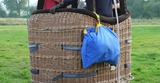Conditional Remix & Share Permitted
CC BY-NC
Rating
0.0 stars

Students use number lines to represent products of a negative integer and a positive integer, and they use patterns to understand products of two negative integers. Students write rules for products of integers.Key ConceptsThe product of a negative integer and a positive integer can be interpreted as repeated addition. For example, 4 &bull; (&ndash;2) = (&ndash;2) + (&ndash;2) + (&ndash;2) + (&ndash;2). On a number line, this can be represented as four arrows of length 2 in a row, starting at 0 and pointing in the negative direction. The last arrow ends at &ndash;8, indicating that 4 &bull; (&ndash;2) = &ndash;8. In general, the product of a negative integer and a positive integer is negative.The product of two negative integers is hard to interpret or visualize. In this lesson, we use patterns to help students see why a negative integer multiplied by a negative integer equals a positive integer. For example, students can compute the products in the pattern below.4 &bull; (&ndash;3) = &ndash;123 &bull; (&ndash;3) = &ndash;92 &bull; (&ndash;3) = &ndash;61 &bull; (&ndash;3) = &ndash;30 &bull; (&ndash;3) = 0They can observe that, as the first factor decreases by 1, the product increases by 3. They can continue this pattern to find these products.&ndash;1 &bull; (&ndash;3) = 3&ndash;2 &bull; (&ndash;3) = 6&ndash;3 &bull; (&ndash;3) = 9In the next lesson, we will prove that the rules for multiplying positive and negative integers extend to all rational numbers, including fractions and decimals.Goals and Learning ObjectivesRepresent multiplication of a negative integer and a positive integer on a number line.Use patterns to understand products of two negative integers.Write rules for multiplying integers.

Subject:
Numbers and Operations
Material Type:
Lesson Plan
09/21/2015Conditional Remix & Share Permitted
CC BY-NC
Rating
0.0 stars

Students solve division problems by changing them into multiplication problems. They then use the relationship between multiplication and division to determine the sign when dividing positive and negative numbers in general.Key ConceptsThe rules for determining the sign of a quotient are the same as those for a product: If the two numbers have the same sign, the quotient is positive; if they have different signs, the quotient is negative. This can be seen by rewriting a division problem as a multiplication of the inverse.For example, consider the division problem &minus;27 &divide; 9. Here are two ways to use multiplication to determine the sign of the quotient:The quotient is the value of x in the multiplication problem 9 &sdot; x = &minus;27. Because 9 is positive, the value of x must be negative in order to get the negative product.The division &minus;27 &divide; 9 is equivalent to the multiplication &minus;27 &sdot; 19. Because this is the product of a negative number and a positive number, the result must be negative.Goals and Learning ObjectivesUse the relationship between multiplication and division to solve division problems involving positive and negative numbers.Understand how to determine whether a quotient will be positive or negative.

Subject:
Numbers and Operations
Material Type:
Lesson Plan
09/21/2015Conditional Remix & Share Permitted
CC BY-NC
Rating
0.0 stars

Students use properties of multiplication to prove that the product of any two negative numbers is positive and the product of a positive number and a negative number is negative.Key ConceptsMultiplication properties can be used to develop the rules for multiplying positive and negative numbers.Students are familiar with the properties from earlier grades:Associative property of multiplication: Changing the grouping of factors does not change the product. For any numbers a, b, and c, (a &sdot; b) &sdot; c = a &sdot; (b &sdot; c).Commutative property of multiplication: Changing the order of factors does not change the product. For any numbers a and b, a &sdot; b = b &sdot; a.Multiplicative identity property of 1: The product of 1 and any number is that number. For any number a, a &sdot; 1 = 1 &sdot; a = a.Property of multiplication by 0: The product of 0 and any number is 0. For any number a, a &sdot; 0 = 0 &sdot; a = 0.Property of multiplication by &minus;1: The product of &minus;1 and a number is the opposite of that number. For any number a, (&minus;1) &sdot; a = &minus;a.Existence of multiplicative inverses: Dividing any number by the same number equals 1. Multiplying any number by its multiplicative inverse equals 1. For every number a &ne; 0, a &divide; a = a &sdot; 1a = 1a &sdot; a = 1.Distributive property: Multiplying a number by a sum is the same as multiplying the number by each term and then adding the products. For any numbers a, b, and c, a &sdot; (b + c) = a &sdot; b + a &sdot; c.In this lesson, students will encounter a proof showing that the product of a positive number and a negative number is negative and two different proofs that the product of two negative numbers is positive. Two alternate proofs are as follows.Proof that the product of two negative numbers is positive:Represent the negative numbers as &minus;a and &minus;b, where a and b are positive.(&minus;a) &sdot; (&minus;b)Original expression= ((&minus;1) &sdot; a) &sdot; ((&minus;1) &sdot; b)&nbsp;&nbsp; Property of multiplication by &minus;1= (&minus;1) &sdot; (a &sdot; (&minus;1)) &sdot; b&nbsp;&nbsp; Associative property of multiplication= (&minus;1) &sdot; ((&minus;1) &sdot; a) &sdot; b&nbsp;&nbsp; Commutative property of multiplication= ((&minus;1) &sdot; (&minus;1)) &sdot; (a &sdot; b)&nbsp;&nbsp; Associative property of multiplication= 1 &sdot; (a &sdot; b)&nbsp;&nbsp; Property of multiplication by &minus;1= a &sdot; b&nbsp;&nbsp; Multiplicative identity property of 1Because a and b are positive, a &sdot; b is positive.Proof that the product of a positive number and a negative number is negative:Let a be the positive number. Let &minus;b be the negative number, where b is positive.a &sdot; (&minus;b)Original expression= a &sdot; ((&minus;1) &sdot; b)&nbsp;&nbsp;&nbsp;&nbsp; Property of multiplication by &minus;1= (a &sdot; (&minus;1)) &sdot; b&nbsp;&nbsp;&nbsp;&nbsp; Associative property of multiplication= ((&minus;1) &sdot; a) &sdot; b&nbsp;&nbsp;&nbsp;&nbsp; Commutative property of multiplication= (&minus;1) &sdot; (a &sdot; b)&nbsp;&nbsp;&nbsp;&nbsp; Associative property of multiplication= &minus;(a &sdot; b)&nbsp;&nbsp;&nbsp;&nbsp; Property of multiplication by &minus;1Because a and b are positive, a &sdot; b is positive, so &minus;(a &sdot; b) must be negative.Goals and Learning ObjectivesReview properties of multiplication.Explain why the product of two negative numbers is positive and the product of a negative number and a positive number is negative.

Subject:
Numbers and Operations
Material Type:
Lesson Plan
09/21/2015Conditional Remix & Share Permitted
CC BY-NC
Rating
0.0 stars

Students critique and improve their work on the Self Check, then work on more addition and subtraction problems.Students solve problems that require them to apply their knowledge of adding and subtracting positive and negative numbers.Key ConceptsTo solve the problems in this lesson, students use their knowledge of addition and subtraction with positive and negative numbers.Goals and Learning ObjectivesUse knowledge of addition and subtraction with positive and negative numbers to write problems that meet given criteria.Assess and critique methods for subtracting negative numbers.Find values of variables that satisfy given inequalities.

Subject:
Numbers and Operations
Material Type:
Lesson Plan
09/21/2015Conditional Remix & Share Permitted
CC BY-NC
Rating
0.0 stars

Students critique and improve their work on the Self Check. They then extend their knowledge with additional problems.Students solve problems that require them to apply their knowledge of multiplying and dividing positive and negative numbers. Students will then take a quiz.Key ConceptsTo solve the problems in the Self Check, students must apply their knowledge of multiplication and division of positive and negative numbers learned throughout the unit.Goals and Learning ObjectivesUse knowledge of multiplication and division of positive and negative numbers to solve problems.

Subject:
Numbers and Operations
Material Type:
Lesson Plan
09/21/2015Conditional Remix & Share Permitted
CC BY-NC
Rating
0.0 stars

Subject:
Numbers and Operations
Material Type:
Lesson Plan
09/21/2015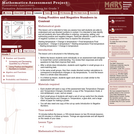Only Sharing Permitted
CC BY-NC-ND
Rating
0.0 stars

This lesson unit is intended to help teachers assess how well students are able to understand and use directed numbers in context. It is intended to help identify and aid students who have difficulties in ordering, comparing, adding, and subtracting positive and negative integers. Particular attention is paid to the use of negative numbers on number lines to explore the structures: starting temperature + change in temperature = final temperature final temperature Đ change in temperature = starting temperature final temperature Đ starting temperature = change in temperature.

Subject:
Mathematics
Numbers and Operations
Material Type:
Assessment
Lesson Plan
Provider:
Shell Center for Mathematical Education
Provider Set:
Mathematics Assessment Project (MAP)
04/26/2013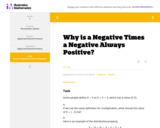Conditional Remix & Share Permitted
CC BY-NC-SA
Rating
0.0 stars

The purpose of this task is for students to understand the reason it makes sense for the product of two negative numbers to be positive. The idea is that if the properties of operations with which we are familiar when we do arithmetic with positive numbers are universal, then we have to define multiplication on signed numbers the way we do. The task only works through a single example, but the argument would work for any two negative numbers.

Subject:
Mathematics
Material Type:
Activity/Lab
Provider:
Illustrative Mathematics
Author:
Illustrative Mathematics